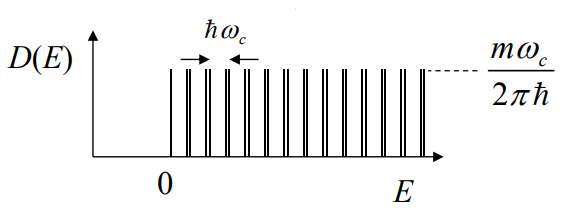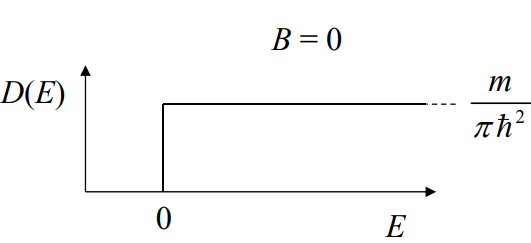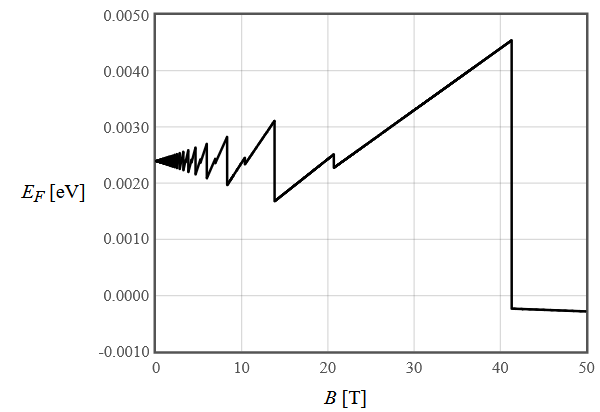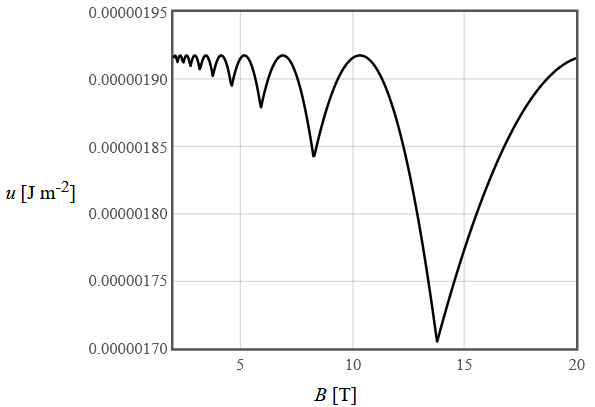## Thermodynamic properties of free electrons in two dimensions in a magnetic field at zero temperature

Often in a calculation, the electron spin can be taken into account by just multiplying the allowed states by a factor of two. Now since we are considering electrons in a magnetic field, the Zeeman effect has to be included in the calculation. The energies of the electrons will be shifted up or down in energy depending on their spin orientation,

$$E_{\text{Zeeman}}= \pm\frac{g\mu_BB}{2}.$$

Here $\mu_B= \frac{e\hbar}{2m}$ is the Bohr magneton and $g$ is the electron spin $g$-factor. Typically, the $g$-factor is approximately 2 so the Zeeman splitting between the up and down orientations is approximately equal to the spacing between the Landau levels, $\hbar\omega_c$.

$$\Delta E_{\text{Zeeman}}=g\mu_BB =g\frac{e\hbar}{2m}B \approx E_{\text{Landau}}= \hbar\omega_c = \hbar\frac{eB}{m}.$$

The resulting two-dimensional density of states consists of two delta functions for each Landau level $\nu$.Plot the 2D density of states.

The integral over each delta function is the degeneracy, $D_0 = \frac{m\omega_c}{h}= \frac{eB}{h}$. The equation for the density of states is,

$$D(E) = \frac{eB}{h}\sum\limits_{\nu=0}^{\infty}\delta\left(E-\hbar\omega_c\left(\nu+\frac{1}{2}-\frac{g}{4}\right)\right)+\delta\left(E-\hbar\omega_c\left(\nu+\frac{1}{2}+\frac{g}{4}\right)\right)$$

At low magnetic fields, the Landau levels are very close together and the concentration of states in an interval $\Delta E$ is $D(E)\Delta E = 2\frac{m\omega_c}{h}\frac{\Delta E}{\hbar \omega_c}$. This is a factor of 2 for spin times the degeneracy of one spin times the number of Landau levels in the energy range $\Delta E$. Thus in the limit of low fields where the Landau levels cannot be distinguished, the density of states becomes constant at $D(E) =\frac{m}{\pi\hbar^2}$ which is the result for free electrons in two dimensions.The internal energy spectral density is the energy times the density of states times the probability that the state is occupied. At low temperature, all of the lower Landau levels will be completely filled and the highest occupied Landau level will be partially filled.Plot $u(E)$.

As the magnetic field increases, the spacing between the Landau levels increases and the degeneracy of each level also increases. This has the consequence that the energy of the highest occupied Landau level increases but the number of electrons in this Landau level decreases as the lower Landau levels can hold more electrons at higher magnetic fields. At some magnetic field, the highest level becomes depopulated and the energy of the highest occupied level jumps down to the next lower Landau level. This makes the Fermi energy and the density of states at the Fermi energy oscillate as the magnetic field is changed. The oscillations are periodic in $1/B$. As a consequence, all of the thermodynamic properties that can be derived from the density of states exhibit oscillations in $1/B$.Plot $E_F$ vs. $B$ Plot $E_F$ vs. $1/B$.

At very high magnetic fields, only the lowest Landau level is occupied. The Landau energy increases linearly with magnetic field but the Zeeman energy decreases linearly with the magnetic field so for $g\approx 2$ these two effects about cancel and the Fermi energy remains about constant.

As B → 0, the Fermi energy converges to the free electron result in two dimensions,

$$E_F=\frac{\pi\hbar^2n}{m}.$$

The internal energy is the integral of the internal energy spectral density. The delta functions in the density of states convert this integral in a sum.

$$u=D_0\hbar\omega_c\left(\sum \limits_{\nu = 0}^{\nu\uparrow_{\text{max}}}\left(\nu+\frac{1}{2}-\frac{g}{4}\right)+\sum \limits_{\nu = 0}^{\nu\downarrow_{\text{max}}}\left(\nu+\frac{1}{2}+\frac{g}{4}\right)\right)+\left(n-\text{Int}\left(\frac{n}{D_0}\right)D_0\right)E_F.$$

The first sum is over the fully occupied Landau levels with spin up electrons and the second sum is over the fully occupied Landau levels with spin down electrons. The last term is the contribution to the energy density due to the partially filled Landau level at the Fermi energy.Plot $u$ vs. $B$ Plot $u$ vs. $1/B$.

As $B\rightarrow0$, the internal energy density approaches the value for free electrons in two dimensions, $u = \frac{nE_F}{2},\quad E_F = \frac{\pi\hbar^2n}{m}$. At very high magnetic fields, only the lowest Landau level is occupied. For $g=2$, the Landau energy that increases linearly with magnetic field cancels the Zeeman that decreases linearly with magnetic field and the internal energy goes to zero.

At zero temperature, the entropy is zero and the internal energy is equal to the Helmholtz free energy. In this limit, the magnetization is the derivative of the internal energy with respect to the magnetic field, $m=-\mu_0\frac{du}{dB}$.Plot $m$ vs. $B$ Plot $m$ vs. $1/B$.

As $B\rightarrow0$, the magnetization oscillates around $m=0$. At very high magnetic fields, only the lowest Landau level is occupied. For $g=2$, the Landau energy that increases linearly with magnetic field cancels the Zeeman that decreases linearly with magnetic field and the magnetization goes to zero. The oscillations in the magnetization are called the De Haas-Van Alphen.

The results presented in this section are summarized in the table of Thermodynamic Properties of free electrons in magnetic field.